New Students Offer - Use Code HELLO

# 10 Important Probability Shortcut & Tricks.• Probability is a measure of how likely an event is to occur.
• Probability is expressed in the form of fractions.
• Favorable number of outcomes
• Total number of outcomes
• Probability ranges between 0 and 1.That means in a total of 5 occurrences, 2 times the occurrences are in my favor.
Favorable outcome is nothing but the condition provided to us in the question.

Example - Consider tossing a fair coin once. What is the probability of getting a heads?
Solution – The first thing is to find the values to keep in the formula.
A total number of outcomes = when you toss a coin once, the possibility is that you may get tails or a heads that give us total 2 possibilities.
The favorable number of outcomes = the condition given to us is to get a heads which are 1 number of time.
Right!!
Now let us put the values in the formulaEasy enough!!
The diagram below can be used as a reference for two and three tosses of a coin respectively.A standard dice has 6 numbers on 6 faces ranging from 1 to 6.
Now if I want to determine the probability of the event of obtaining a number 4 in one roll of a dice.
The total number of outcomes = when you roll a dice once, the possibility is that you may get a number 1 or 2 or 3 or 4 or 5 or 6, which gives us a total number of 6 possibilities.
The favorable number of outcomes = the condition given to us is to get a number 4 which is 1 number of time.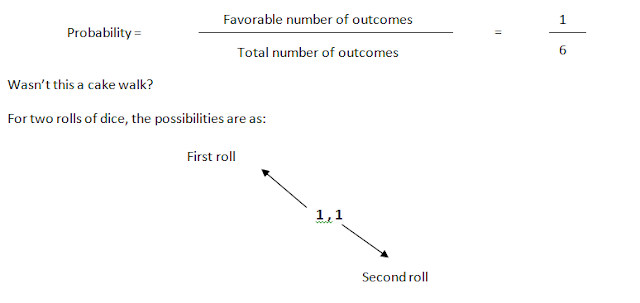1,1 1,2 1,3 1,4 1,5 1,6 2,1 2,2 2,3 2,4 2,5 2,6 3,1 3,2 3,3 3,4 3,5 3,6 4,1 4,2 4,3 4,4 4,5 4,6 5,1 5,2 5,3 5,4 5,5 5,6 6,1 6,2 6,3 6,4 6,5 6,6
Example - Consider rolling a standard dice twice. What is the probability of getting a prime number in the first roll?

Solution – The first thing that we do is find the total number of possibilities. In the question, we need to roll the dice twice. From the above table, we get the total number of 36 possibilities.
To find the favorable number of outcomes, we read the condition which is to get a prime number in the first roll. Among the numbers 1, 2, 3, 4, 5, 6 on the dice, the prime numbers are 2, 3 and 5.
With these numbers on the first roll we have

 2,1 2,2 2,3 2,4 2,5 2,6 3,1 3,2 3,3 3,4 3,5 3,6 5,1 5,2 5,3 5,4 5,5 5,6
these many possibilities. Total 18
Keeping the values in the formulaFor the sum related questions on two rolls of a dice, try and remember this technique.
Now, what does this mean?
The lowest sum after the two rolls of a dice is 2 from (1, 1) while the highest sum is 12 from (6, 6), which means the total number of possibilities of getting a sum of 2 or 12 is 1 (which is the number on their right most side).
Similarly, the number of possibilities of getting a sum of 3 or 11 is 2 (which is the number on their right most side) and so on.
Just a look at the above diagram will make the sum related problems of a dice easy for you.

Example – What is the probability of getting a sum 9 from two rolls of a dice?
Solution – The first thing that we do is find the total number of possibilities which is 36.
Now to get a sum of 9, from the above table, the number on the rightmost side of the row having number 9 is 4. This means 4 is the favorable number of outcomes.
Cross-check – To get sum 9, the possibilities are (4,5), (5,4), (3,6), (6,3), 4 in total, thus justifying the authenticity of the table.
Now, Putting values in the formula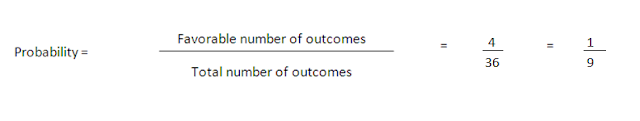Few practice problems:

1.  Two dice are tossed. The probability that a total score is a prime number is?
2.  Two dice are thrown simultaneously. What is the probability of getting two numbers whose product is even?
3.  In a simultaneous throw of a pair of dice, find the probability of getting equal numbers.

### Another important set of question in probability consists of cards. The hierarchy below represents the types of cards in a deck.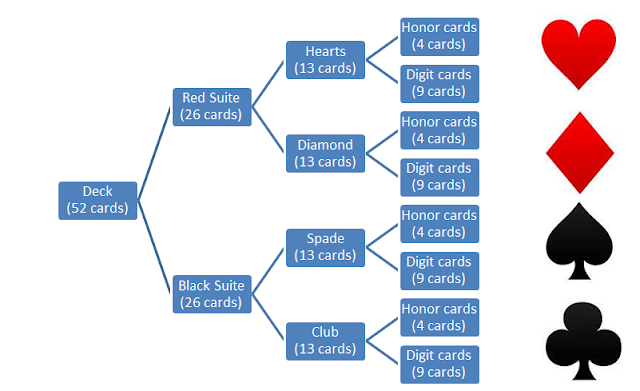Digit cards – 2 to 10 (total 9 cards of one type and there are 4 types of cards, which gives us a total of 9 x 4 = 36 digit cards in a deck)
Honor cards – Ace, King, Queen, Jack (4 of each type and there are 4 types, which gives us a total of 4 x 4 = 16 honor cards in a deck)
When we talk about the favorable condition (given to us in the question) in the problems related to cards, we need to have our concepts crystal clear of COMBINATIONS.

#### Let’s work with examples

Example – What is the probability of picking up an ace in a deck of 52 cards?Hope the concept is clear by now. How about we solve a question together?

Question - From a pack of 52 cards, two cards are drawn together at random. What is the probability of both the cards being kings?

Solution –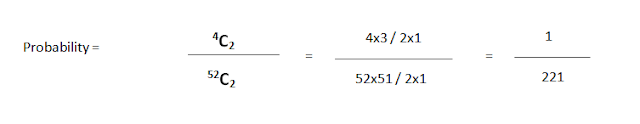Question – What is the probability of drawing a card from a deck which is either a king or a Diamond card?

Solution –You might be wondering about the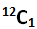as Diamond cards are total 13 in number. Well guys, when we selected a king card, which are 4 in number, the set of 4 also had a diamond card in it, Thus leaving 12 diamond cards for us to select from.
We can also select a diamond card first and then a king card. The equation then would look likeThe answer in both the cases will be similar.
Few practice problems:

1. Two cards are drawn together from a pack of 52 cards. The probability that one is a spade and one is a heart, is.
2. One card is drawn at random from a pack of 52 cards. What is the probability that the card drawn is an Honor card (Jack, Queen, and King only)?
3. If three cards are drawn at random from a well shuffled deck. What is the probability of picking out 2 Jacks of red color and 1 black-color digit card?
4. 2 cards are drawn at random from a deck. Find the probabilities of picking out

• Exactly 2 Queens.
• At least one Queen.
• No Queen.
•  At most 1 Queen.

Last (but not the least) are the questions asking you the probabilities with the condition when a ball is picked from a bag.
Let’s start the concept with an example.

Example – What is the probability of picking out one white ball from a bag that has 4 white balls in it?
Solution – The bag contains white and white balls only. So no matter how many times you take out a ball it is going to be white undoubtedly. Therefore, the probability of the event happening becomes 1.

Another Example,

Example – What is the probability of picking out a blue ball from a bag that contains 7 black balls?
Solution –I hope the answer’s pretty easy. 0 (Isn’t it!)

Example – A bag contains 6 Blue, 4 White balls. If 2 balls are drawn at a time, find the probability of picking out balls of different colors.
Solution –As there are 2 colors and 2 balls are drawn then one of them must be blue while the other one must be white. (Clear!)

ThereforeWhat if in the same question, the condition is changed to “If 2 balls are drawn at a time, find the probability of picking out balls of same color.”

Then, as there are 2 colors and 2 balls are drawn then both the balls drawn are either white in color or blue in color. (Clear!). Therefore, the equation changes as followsLet us discuss one other example.

Example – A bag contains 5 White, 2 Red, 3 Black balls. If 2 balls are drawn at a time, find the probability of picking out at least one white ball.

Solution – The word “at least” implies the picking of either 1 white ball or both white balls. Now, if there is 1 white ball in the selection that means other one picked ball is of other color (meaning red and black in this case). And 2 white balls means there cannot be any other colored ball.

Therefore, the equation becomesTrick – What if rather than calculating all the possibilities of at least (minimum 1 ball), I simply consider the possibility of no white balls and subtracted it from 1(highest probability of an event happening)

If there is no white ball, this means all the two balls are selected from 2 red and 3 black balls, means total of 5 balls.

Selecting 2 balls from 5 gives me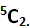And the total number of possibilities is selecting two balls from total number of balls (in this case 10 balls), which isSo, the probability becomesThe calculation of which gives meNow, don’t forget the step where we promised to subtract it from highest probability 1.

So,This is the final probability.
Isn’t it the same to what we calculated earlier? The trick takes some time but with some practice, you will get a hang of it. The trick with “at least” can be applied to the problems with deck of cards too.

Few practice problems:

1. A bag contains 2 red, 3 green and 2 blue balls. Two balls are drawn at random. What is the probability that none of the balls drawn is blue?
2. A bag contains 4 white, 5 red and 6 blue balls. Three balls are drawn at random from the bag. The probability that all of them are red, is?

There are a lot of questions of probability available on the various sources. Give the calculations mentioned above a chance, I promise no one (and I mean no one) would be able to stop you from scoring 100% marks in PROBABILITY.

**Good Luck**